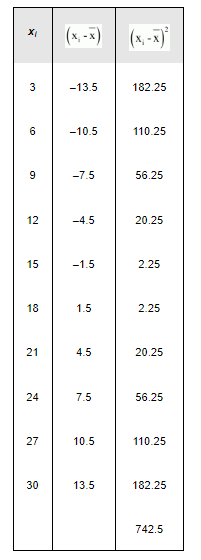# Find the mean and variance for the first 10 multiples of 3

Question:

Find the mean and variance for the first 10 multiples of 3

Solution:

The first 10 multiples of 3 are

3, 6, 9, 12, 15, 18, 21, 24, 27, 30

Here, number of observations, = 10

Mean, $\bar{x}=\frac{\sum_{i=1}^{10} x_{i}}{10}=\frac{165}{10}=16.5$

The following table is obtained.Variance $\left(\sigma^{2}\right)=\frac{1}{n} \sum_{i=1}^{10}\left(x_{i}-\bar{x}\right)^{2}=\frac{1}{10} \times 742.5=74.25$